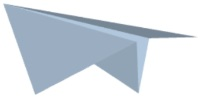## Lesson 5: An Engineering Introduction to Machines–Inclined Planes (January 8 and 9)

Objectives: (1) To introduce students to the basic concepts of machines, as used by engineers; and (2) to specifically learn the most basic principles of the INCLINED PLANE.

## Lesson 4: An Engineering Introduction to Machines–Pulleys (December 13 & 14)

Objectives: (1) To introduce students to the basic concepts of machines, as used by engineers; and (2) to specifically learn the most basic principles of the PULLEY.

## Lesson 3: An Engineering Introduction to Machines–Gears (December 11 & 12)

Objectives: (1) To introduce students to the basic concepts of machines, as used by engineers; and (2) to specifically learn the most basic principles of gears.

## Lesson 2: An Engineering Introduction to Machines–Wheel and Axle (December 7 & 10)

Objectives: (1) To introduce students to the basic concepts of machines, as used by engineers; and (2) to specifically learn the most basic principles of the wheel and axle.

## Lesson 1 An Engineering: Introduction to Machines–Levers (December 5 & 6)

Objectives: (1) To introduce students to the basic concepts of machines, as used by engineers; and (2) to specifically learn the principles of levers.

## Lesson 8 on Aeronautical Engineering: Paper Airplane, Final Design Review (November 29 & 30)

1. The Paper Plane Contest will take place next class in the courtyard outside this building. Please be familiar with the contest rules before then.
2. Each team will make their paper airplanes on the morning of the contest, using paper supplied by the teacher.
3. On the morning of the competition, each team will be supplied with paper and an agenda to prepare for the competition.

## Lesson 7 on Aeronautical Engineering: Paper Airplane Design (November 27 & 28)

Objectives: (1) To introduce students to the basic concepts of aviation, which will be illustrated through the construction of paper airplanes; and (2) to help students learn to follow instructions in approaching assignments.

## Lesson 6 on Aeronautical Engineering: Paper Airplane Design (November 16 and 26)

Important:  Read all instructions before proceeding.

Objectives: (1) To reinforce information students are learning about the basic concepts of aviation, which will be illustrated through the design of a paper airplane in this lesson; and (2) To demonstrate that engineering is a combination of science with learning from trial and error.

## Lesson 5 on Aeronautical Engineering: Videos on Paper Airplane Construction (November 14 and 15)

Watch the video clips as you answer the questions in the Assignment below.  You will need to watch the video clips in order to fill in the blanks.

Objectives: (1) To introduce students to the basic concepts of aviation, which will be illustrated through the construction of paper airplanes in subsequent lessons; and (2) To improve listening and note taking skills  while watching the videos in class.